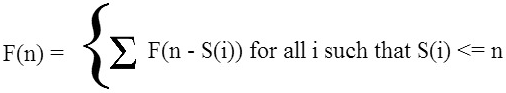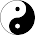Wednesday, October 16, 2013

A closer look at Coin Change

Coin change has been a very famous computing problem which can be easily solved by Dynamic programming.

So what is coin change ?
If we want to make change for N cents, and we have infinite supply of each of$S = \{ S_1, S_2, \ldots, S_m \}$ valued coins, how many ways can we make the change? (The order does not matter.)

For example, for N = 4,S = {1,2,3}, there are 7 possible solutions: {1,1,1,1},{1,1,2}, {1, 2, 1}, {2, 1, 1}, {2,2},{1,3}, {3, 1}.

So how to compute the number of ways efficiently?

Lets assume you know the number of ways to generate the sum 1, 2, 3 , can you find the number of  ways to generate 4?

Yes, you can, indeed you have just broken the problem into the subproblems, a basic paradigm of the dynamic programming.

Let assume F(n) denotes the number of solutions possible.
So F(4) = F(3) + F(2) + F(1)

In general if S[i] denotes the denominations of the coins thenThe below piece of code generates the number of combinations for a particular sum.
```int coin_change_infinite(int s[], int n, int val) {
int * a = (int *) calloc(1, sizeof(int) * (val + 1));
int res;
sort(s, s + n);
a = 1;
for(int i = 1; i <= val; i++)
for(int j = 0; s[j] <= i && j < n; j++)
a[i] += a[i - s[j]];
res = a[val];
free(a);
return res;
}```

Overall running time for the algorithm is O(n*c) (n is the value for which the denominations needs to be made and c is the types of denominations available.) and space complexity is O(n)

1.1.